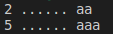# Regular Expressions in Python

## Regular Expressions in Python

In this article, I am going to discuss Regular Expressions in Python with examples. Please read our previous article where we discussed Exception Handling in Python. As part of this article, we are going to discuss the following pointers in details.

1. What are Regular Expressions?
2. When should we choose Regular Expressions in Python?
3. Application areas of Regular Expressions
4. Character Classes
5. Predefined Character classes in Python
6. Quantifiers in Python
##### What are Regular Expressions?

A regular expression is a sequence of characters that define a search pattern in theoretical computer science and formal language.

##### When should we choose Regular Expressions in Python?

If we want to represent a group of Strings according to a particular format/pattern then we should go for Regular Expressions. Regular Expression is a declarative mechanism to represent a group of Strings according to a particular format/pattern.

Example 1: We can write a regular expression to represent all mobile numbers

Example 2: We can write a regular expression to represent all mail ids.

##### Application areas of Regular Expressions
1. To develop validation frameworks/validation logic.
2. To develop Pattern matching applications (ctrl-f in windows, grep in UNIX, etc)
3. To develop Translators like compilers, interpreters, etc
4. To develop digital circuits
5. To develop communication protocols like TCP/IP, UDP, etc

We can develop Regular Expression Based applications by using the python module: re. This module contains several inbuilt functions to use Regular Expressions very easily in our applications.

##### compile():

re module contains a compile() function to compile a pattern into RegexObject. It returns a pattern object, if the pattern is valid, which can be used for searching.

pattern_obj = re.compile(“<pattern>”)

##### finditer():

This method when called upon the pattern object will return an Iterator object, which yields Match object for every Match.

matcher = pattern_obj.finditer(<string to be searched for match>)

###### Program: importing re module and working with methods (demo1.py)
```import re
count=0
pattern=re.compile("ab")
matcher=pattern.finditer("abaababa")
for match in matcher :
count+=1
print(match.start(),"...",match.end(),"...", match.group())
print("The number of occurrences: ", count)```

Output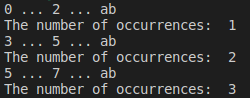###### Note:
1. start(): This method when called on the match object will returns the start index of the match.
2. end(): This method when called on the match object will return end+1 index of the match.
3. group(): This method will return the matched string

Note: Instead of creating a pattern object and then searching for a pattern, we can directly pass patterns as arguments to finditer() function.

matcher = re.finditer(<pattern>, <string to be searched for match>)

###### Program: pattern as arguments to finditer() function (demo2.py)
```import re
count=0
matcher=re.finditer("ab", "abaababa")
for match in matcher :
count+=1
print(match.start(),"...",match.end(),"...", match.group())
print("The number of occurrences: ", count)```

Output:##### CHARACTER CLASSES:

We can use character classes to search for a group of characters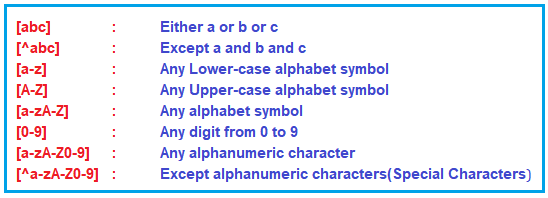Program: Character Classes (demo3.py)

```import re
matcher=re.finditer("[abc]","a7b@k9z")
for match in matcher :
print(match.start(),"......",match.group())```

Output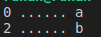###### Program: demo4.py
```import re
matcher=re.finditer("[^abc]","a7b@k9z")
for match in matcher :
print(match.start(),"......",match.group())```

Output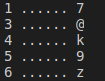###### Program: demo5.py
```import re
matcher=re.finditer("[a-z]","a7b@k9z")
for match in matcher :
print(match.start(),"......",match.group())```

Output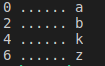###### Program: demo6.py
```import re
matcher=re.finditer("[0-9]","a7b@k9z")
for match in matcher:
print(match.start(),"......",match.group())```

Output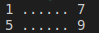###### Program: demo7.py
```import re
matcher=re.finditer("[a-zA-Z0-9]","a7b@k9z")
for match in matcher:
print(match.start(),"......",match.group())```

Output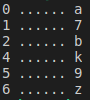###### Program: demo8.py
```import re
matcher=re.finditer("[^a-zA-Z0-9]","a7b@k9z")
for match in matcher:
print(match.start(),"......",match.group())```

Output: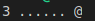##### Predefined Character classes in Python: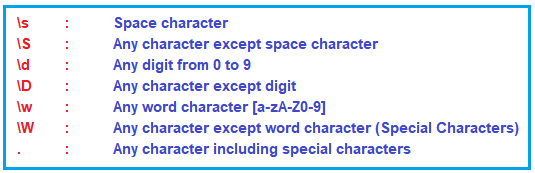Program: demo9.py

```import re
matcher=re.finditer("\s","a7b @k9z")
for match in matcher:
print(match.start(),"......",match.group())```

Output: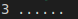###### Program: demo10.py
```import re
matcher=re.finditer("\S","a7b @k9z")
for match in matcher:
print(match.start(),"......",match.group())```

Output: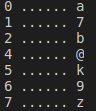###### Program: demo11.py
```import re
matcher=re.finditer("\d","a7b @k9z")
for match in matcher:
print(match.start(),"......",match.group())```

Output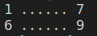###### Program: demo12.py
```import re
matcher=re.finditer("\D","a7b @k9z")
for match in matcher:
print(match.start(),"......",match.group())```

Output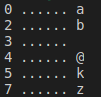###### Program: demo13.py
```import re
matcher=re.finditer("\w","a7b @k9z")
for match in matcher:
print(match.start(),"......",match.group())```

Output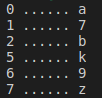###### Program: demo14.py
```import re
matcher=re.finditer("\W","a7b @k9z")
for match in matcher:
print(match.start(),"......",match.group())```

Output: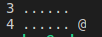###### Program: demo15.py
```import re
matcher=re.finditer(".","a7b @k9z")
for match in matcher:
print(match.start(),"......",match.group())```

Output: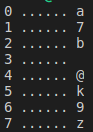##### Quantifiers in Python:

A quantifier has the form {m,n} where m and n are the minimum and maximum times the expression to which the quantifier applies must match. We can use quantifiers to specify the number of occurrences to match.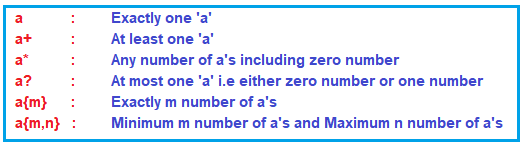###### Program: demo16.py
```import re
matcher=re.finditer("a","abaabaaab")
for match in matcher:
print(match.start(),"......",match.group())```

Output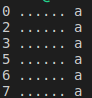###### Program: demo17.py
```import re
matcher=re.finditer("a+","abaabaaab")
for match in matcher:
print(match.start(),"......",match.group())```

Output: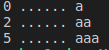###### Program: demo18.py
```import re
matcher=re.finditer("a*","abaabaaab")
for match in matcher:
print(match.start(),"......",match.group())```

Output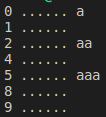###### Program: demo19.py
```import re
matcher=re.finditer("a?","abaabaaab")
for match in matcher:
print(match.start(),"......",match.group())```

Output: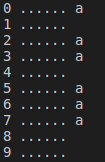###### Program: demo20.py
```import re
matcher=re.finditer("a{3}","abaabaaab")
for match in matcher:
print(match.start(),"......",match.group())```

Output: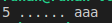###### Program: demo21.py
```import re
matcher=re.finditer("a{2,4}","abaabaaab")
for match in matcher:
print(match.start(),"......",match.group())```

Output